Home Practice
For learners and parents For teachers and schools
Textbooks
Full catalogue
Pricing SupportLog in

We think you are located in United States. Is this correct?

# 7.4 The mid-point theorem

## Proving the mid-point theorem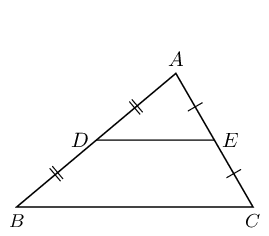1. Draw a large scalene triangle on a sheet of paper.

2. Name the vertices $$A$$, $$B$$ and $$C$$. Find the mid-points ($$D$$ and $$E$$) of two sides and connect them.

3. Cut out $$\triangle ABC$$ and cut along line $$DE$$.

4. Place $$\triangle ADE$$ on quadrilateral $$BDEC$$ with vertex $$E$$ on vertex $$C$$. Write down your observations.

5. Shift $$\triangle ADE$$ to place vertex $$D$$ on vertex $$B$$. Write down your observations.

6. What do you notice about the lengths $$DE$$ and $$BC$$?

7. Make a conjecture regarding the line joining the mid-point of two sides of a triangle.

## Worked example 8: Mid-point theorem

Prove that the line joining the mid-points of two sides of a triangle is parallel to the third side and equal to half the length of the third side.### Extend $$DE$$ to $$F$$ so that $$DE=EF$$ and join $$FC$$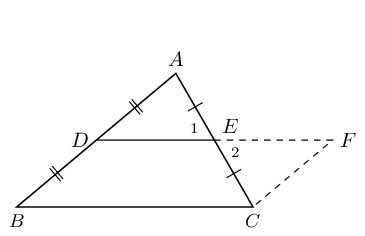### Prove $$BCFD$$ is a parallelogram

In $$\triangle EAD$$ and $$\triangle ECF$$:

$\begin{array}{rll} \hat{E}_{1} & = \hat{E}_{2}& \text{(vert opp }\angle \text{s} = \text{)}\\ AE & = CE & \text{ (given)} \\ DE & = EF & \text{ (by construction)} \\ \therefore \triangle EAD & \equiv \triangle ECF & \text{(SAS)} \\ \therefore A\hat{D}E & = C\hat{F}E & \end{array}$

But these are alternate interior angles, therefore $$BD\parallel FC$$

$\begin{array}{rll} BD & = DA & \text{(given)}\\ DA & = FC & (\triangle EAD\equiv \triangle ECF)\\ \therefore BD& = FC & \end{array}$ $\therefore BCFD \text{ is a parallelogram } \text{(one pair opp. sides } = \text{ and }\parallel \text{)}$

Therefore $$DE\parallel BC$$.

We conclude that the line joining the two mid-points of two sides of a triangle is parallel to the third side.

### Use properties of parallelogram $$BCFD$$ to prove that $$DE=\frac{1}{2}BC$$

$\begin{array}{rll} DF & = BC & \text{(opp sides of } \parallel \text{m)} \\ \text{and } DF & = 2\left(DE\right) & \text{(by construction)} \\ \therefore 2DE & = BC & \\ \therefore DE & = \frac{1}{2}BC & \end{array}$

We conclude that the line joining the mid-point of two sides of a triangle is equal to half the length of the third side.

Converse

The converse of this theorem states: If a line is drawn through the mid-point of a side of a triangle parallel to the second side, it will bisect the third side.

temp text

You can use GeoGebra to show that the converse of the mid-point theorem is true.

Textbook Exercise 7.7

Points $$C$$ and $$A$$ are the mid-points on lines $$BD$$ and $$BE$$. Study $$\triangle EDB$$ carefully. Identify the third side of this triangle, using the information as shown, together with what you know about the mid-point theorem. Name the third side by its endpoints, e.g., $$FG$$.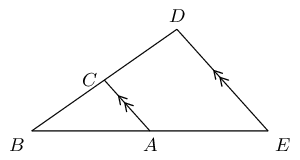The red line, $$ED$$ or $$DE$$, indicates the third side of the triangle. According to the mid-point theorem, the line joining the mid-points of two sides of a triangle is parallel to the third side of the triangle.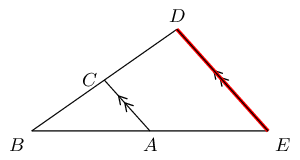Points $$R$$ and $$P$$ are the mid-points on lines $$QS$$ and $$QT$$. Study $$\triangle TSQ$$ carefully. Identify the third side of this triangle, using the information as shown, together with what you know about the mid-point theorem. Name the third side by its endpoints, e.g., $$FG$$.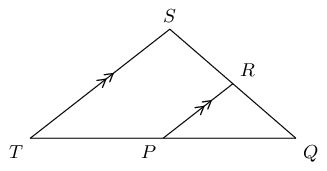The red line, $$TS$$ or $$ST$$, indicates the third side of the triangle. According to the mid-point theorem, the line joining the mid-points of two sides of a triangle is parallel to the third side of the triangle.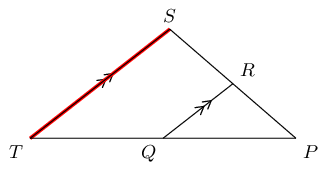Points $$C$$ and $$A$$ are given on the lines $$BD$$ and $$BE$$. Study the triangle carefully, then identify and name the parallel lines.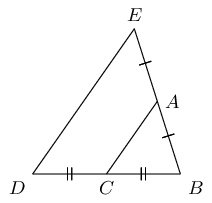The lines $$ED$$ and $$AC$$ are parallel according to the mid-point theorem because $$AC$$ is bisecting the lines $$EB$$ and $$DB$$.

Points $$R$$ and $$P$$ are given on the lines $$QS$$ and $$QT$$. Study the triangle carefully, then identify and name the parallel lines.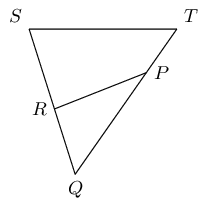The lines $$TS$$ and $$PR$$ are not parallel according to the mid-point theorem because line $$PR$$ does not bisect $$TQ$$ and $$SQ$$. Therefore there are no parallel lines in the triangle.

The figure below shows a large triangle with vertices $$A$$, $$B$$ and $$D$$, and a smaller triangle with vertices at $$C$$, $$D$$ and $$E$$. Point $$C$$ is the mid-point of $$BD$$ and point $$E$$ is the mid-point of $$AD$$.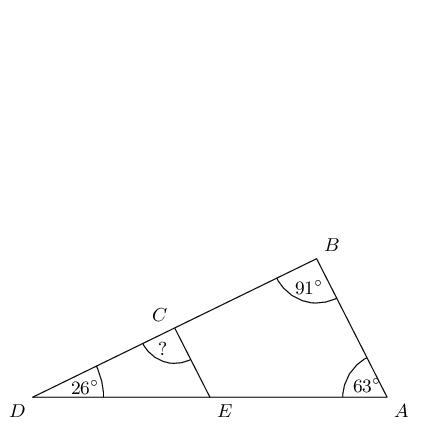Three angles are given: $$\hat{A} = 63^{\circ}$$, $$\hat{B} = 91^{\circ}$$ and $$\hat{D} = 26^{\circ}$$; determine the value of $$D\hat{C}E$$.

\begin{align*} AB & \parallel EC \qquad \text{(Midpt Theorem)} \\ \therefore D\hat{C}E & = \hat{B} \qquad \text{(corresp } \angle \text{s; } AB \parallel EC\text{)} \\ D\hat{C}E & = 91^{\circ} \end{align*}

The two triangles in this question are similar triangles. Complete the following statement correctly by giving the three vertices in the correct order (there is only one correct answer).

$$\triangle DEC \enspace|||\enspace \triangle ?$$

Angle $$D$$ corresponds to angle $$D$$; angle $$E$$ corresponds to angle $$A$$; and angle $$C$$ corresponds to angle $$B$$. Therefore, $$\triangle DEC \enspace|||\enspace \triangle DAB$$.

The figure below shows a large triangle with vertices $$G$$, $$H$$ and $$K$$, and a smaller triangle with vertices at $$J$$, $$K$$ and $$L$$. Point $$J$$ is the mid-point of $$HK$$ and point $$L$$ is the mid-point of $$GK$$.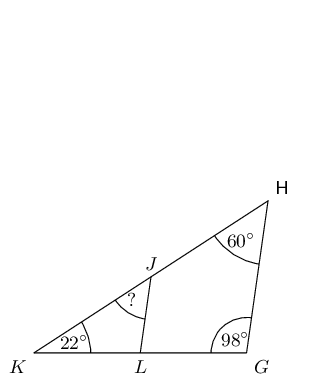Three angles are given: $$\hat{G} = 98^{\circ}$$, $$\hat{H} = 60^{\circ}$$, and $$\hat{K} = 22^{\circ}$$; determine the value of $$K\hat{J}L$$.

\begin{align*} GH & \parallel LJ \qquad \text{(Midpt Theorem)} \\ \therefore K\hat{J}L & = \hat{H} \qquad \text{(corresp } \angle \text{s; } GH \parallel LJ\text{)}\\ K\hat{J}L & = 60^{\circ} \end{align*}

The two triangles in this question are similar triangles. Complete the following statement correctly by giving the three vertices in the correct order (there is only one correct answer).

$$\triangle HKG \enspace|||\enspace \triangle ?$$

Angle $$H$$ corresponds to angle $$J$$; angle $$K$$ corresponds to angle $$K$$ ; and angle $$G$$ corresponds to angle $$L$$. Therefore, $$\triangle HKG \enspace|||\enspace \triangle JKL$$.

Consider the triangle in the diagram below. There is a line crossing through a large triangle. Notice that some lines in the figure are marked as equal to each other. One side of the triangle has a given length of 3. Some information is also given about the lengths of other lines along the edges of the triangle.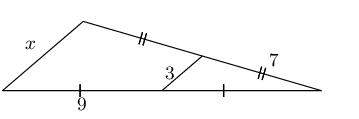Determine the value of $$x$$.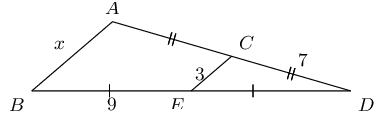From the mid-point theorem we know:

\begin{align*} AB & = 2 \times CE \\ x & = 2(3) \\ & = 6 \end{align*}

Consider the triangle in the diagram below. There is a line crossing through a large triangle. Notice that some lines in the figure are marked as equal to each other. One side of the triangle has a given length of 6.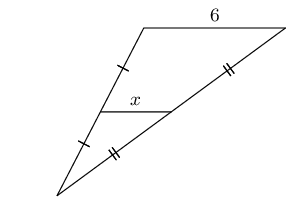Determine the value of $$x$$.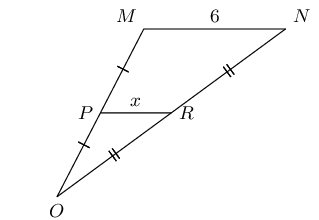From the mid-point theorem we know:

\begin{align*} MN & = 2 \times PR \\ \left( 6 \right) & = 2x \\ \frac{1}{2} \left( 6 \right) & = x \\ 3 & = x \end{align*}

In the figure below, $$VW \parallel ZX$$, as labelled. Furthermore, the following lengths and angles are given: $$VW = 12$$; $$ZX = 6$$; $$XY = \text{5,5}$$; $$YZ = 5$$ and $$\hat{V} = 59^{\circ}$$. The figure is drawn to scale.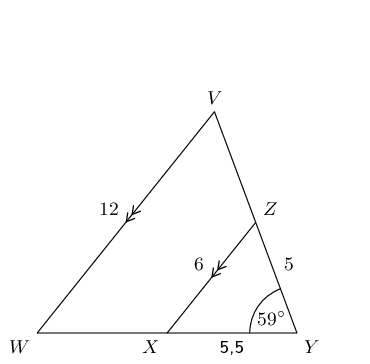Determine the length of $$WY$$.

$$X$$ is the mid-point of $$WY$$ and $$Z$$ is the mid-point of $$VY$$ ($$VW \parallel ZX$$, also it is given that $$XZ = \frac{1}{2}VW$$).

\begin{align*} WY & = 2 \times XY \qquad \text{definition of mid-point} \\ & = 2(\text{5,5}) \\ & = \text{11} \end{align*}

In the figure below, $$VW \parallel ZX$$, as labelled. Furthermore, the following lengths and angles are given: $$VW = 4$$; $$ZX = 2$$; $$WX = 4$$; $$YZ = \text{3,5}$$ and $$\hat{Y} = 30^{\circ}$$. The figure is drawn to scale.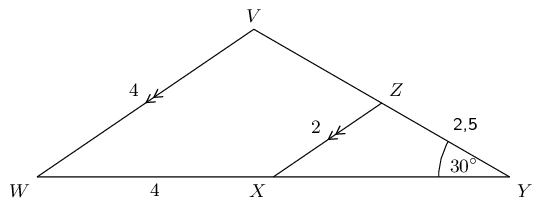Determine the length of $$XY$$.

$$X$$ is the mid-point of $$WY$$ and $$Z$$ is the mid-point of $$VY$$ ($$VW \parallel ZX$$, also it is given that $$XZ = \frac{1}{2}VW$$).

\begin{align*} XY & = WX \qquad \text{definition of mid-point} \\ & = \text{4} \end{align*}

Find $$x$$ and $$y$$ in the following: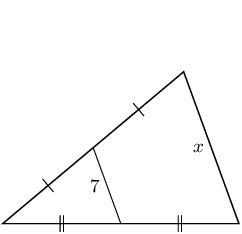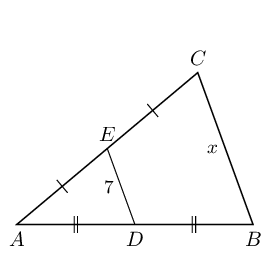From the mid-point theorem we know:

\begin{align*} BC & = 2 \times DE \\ x & = 2(7) \\ & = 14 \end{align*}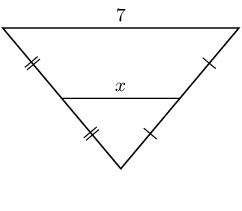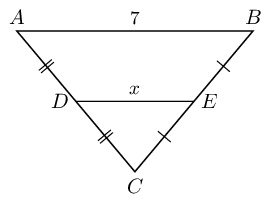From the mid-point theorem we know:

\begin{align*} AB & = 2 \times DE \\ 7 & = 2x \\ \text{3,5} & = x \end{align*}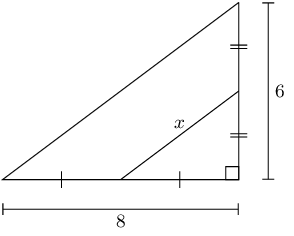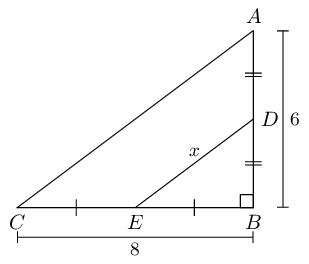We can use the theorem of Pythagoras to find $$AC$$:

\begin{align*} AC^{2} & = BC^{2} + AB^{2} \\ & = (8)^{2} + (6)^{2} \\ & = 64 + 36 \\ & = 100 \\ AC & = 10 \end{align*}

From the mid-point theorem we know:

\begin{align*} AC & = 2 \times DE \\ 10 & = 2x \\ \text{5} & = x \end{align*}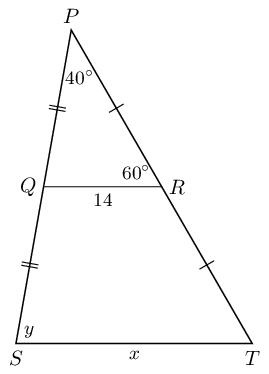From the mid-point theorem we know:

\begin{align*} ST & = 2 \times QR \\ x & = 2(14) \\ & = 28 \end{align*}

To find $$y$$ we note the following:

• $$P\hat{Q}R = 180° - 60° - 40° = 100°$$ (sum of $$\angle$$s in $$\triangle$$).

• From the mid-point theorem we also know that $$QR \parallel ST$$.

Therefore $$y = 100°$$ (corresp $$\angle$$s; $$QR \parallel ST$$).

The final answer is: $$x = 28\text{ units}$$ and $$y = 100°$$.

In the following diagram $$PQ = \text{2,5}$$ and $$RT = \text{6,5}$$.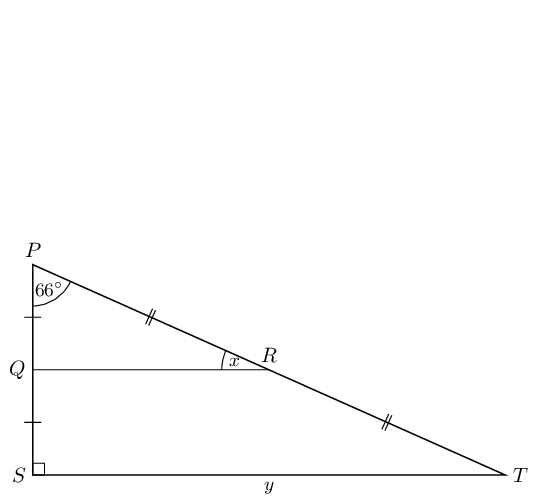From the mid-point theorem we know that $$QR \parallel ST$$. Therefore $$P\hat{Q}R = P\hat{S}T = 90°$$ (corresp $$\angle$$s; $$QR \parallel ST$$).

Therefore $$x = 180° - 90° - 66° = 24°$$ (sum of $$\angle$$s in $$\triangle$$).

To find $$y$$ we note that $$PQ + QS = PS$$ and $$PQ = QS$$, therefore $$PS = 2PQ$$. Similarly $$PT = 2RT$$.

We can use the theorem of Pythagoras to find $$ST$$:

\begin{align*} ST^{2} & = PS^{2} + PT^{2} \\ & = 2PQ + 2RT \\ & = (2(\text{2,5}))^{2} + (2(\text{6,5}))^{2} \\ & = \text{25} + \text{169} \\ & = 194 \\ ST & = \text{13,93} \end{align*}

Therefore: $$x = 24°$$ and $$y = \text{13,93}$$.

Show that $$M$$ is the mid-point of $$AB$$ and that $$MN = RC$$.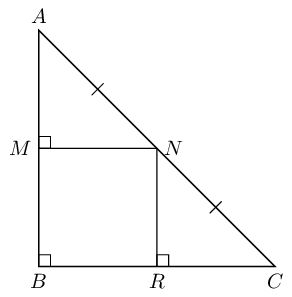We are given that $$AN = NC$$.

We are also given that $$\hat{B} = \hat{M} = 90°$$, therefore $$MN \parallel BR$$ ($$\hat{B}$$ and $$\hat{M}$$ are equal, corresponding angles).

Therefore $$M$$ is the mid-point of $$AB$$ (converse of mid-point theorem).

Similarly we can show that $$R$$ is the mid-point of $$BC$$.

We also know that $$MN = BR$$ ($$MB \parallel NR$$ and parallel lines are a constant distance apart).

But $$BR = RC$$ ($$R$$ is the mid-point of $$BC$$), therefore $$MN = RC$$.

In the diagram below, $$P$$ is the mid-point of $$NQ$$ and $$R$$ is the mid-point of $$MQ$$. The segment inside of the large triangle is labelled with a length of $$-2a + 4$$.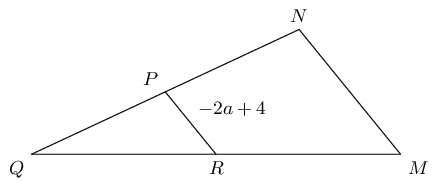Calculate the value of $$MN$$ in terms of $$a$$.

Use the mid-point theorem to fill in known information on the diagram: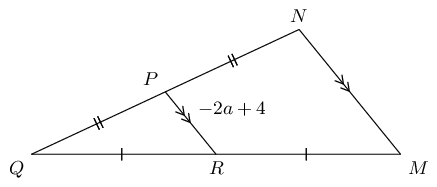Remember that the mid-point theorem tells us that the segments $$MN$$ and $$PR$$ have a ratio of $$2 : 1$$ ($$MN$$ is twice as long as $$PR$$).

\begin{align*} MN & = 2 \times PR\\ & = 2 \left( -2a + 4 \right) \\ & = -4a + 8 \end{align*}

The final answer is $$MN = -4a + 8$$ (twice as long as $$PR$$).

You are now told that $$MN$$ has a length of $$\text{18}$$. What is the value of $$a$$? Give your answer as a fraction.

\begin{align*} - 4 a + 8 & = \text{18} \\ - 4 a & = 10 \\ \left( - \frac{1}{4} \right) \left( - 4 a \right) & = \left( 10 \right) \left( - \frac{1}{4} \right) \\ a & = - \frac{5}{2} \end{align*}

In the diagram below, $$P$$ is the mid-point of $$NQ$$ and $$R$$ is the mid-point of $$MQ$$. One side of the triangle has a given length of $$\dfrac{2 a}{3} + 4$$.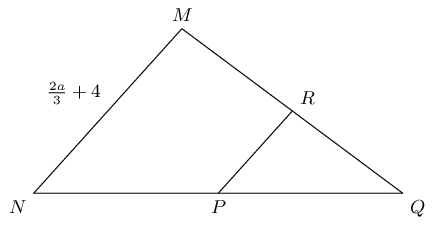Find the value of $$PR$$ in terms of $$a$$.

Use the mid-point theorem to fill in known information on the diagram: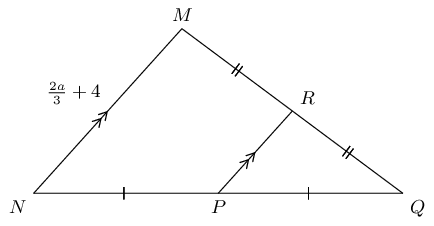Remember that the mid-point theorem tells us that the segments $$MN$$ and $$PR$$ have a ratio of $$2 : 1$$ ($$MN$$ is twice as long as $$PR$$).

\begin{align*} MN & = 2 \times PR \\ \left( \frac{2 a}{3} + 4 \right) & = 2 \left(PR\right) \\ \frac{1}{2} \left( \frac{2 a}{3} + 4 \right) & = PR \\ \frac{a}{3} + 2 & = PR \end{align*}

You are now told that $$PR$$ has a length of $$\text{8}$$. What is the value of $$a$$?

\begin{align*} \frac{a}{3} + 2 & = \text{8} \\ \frac{a}{3} & = 6 \\ \left( 3 \right) \left( \frac{a}{3} \right) & = \left( 6 \right) \left( 3 \right) \\ a & = 18 \end{align*}

The figure below shows $$\triangle ABD$$ crossed by $$EC$$. Points $$C$$ and $$E$$ bisect their respective sides of the triangle.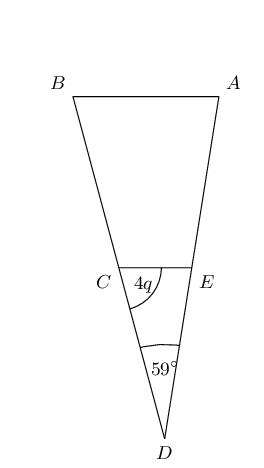The angles $$\hat{D} = 59^{\circ}$$ and $$E\hat{C}D = 4 q$$ are given; determine the value of $$\hat{A}$$ in terms of $$q$$.

We note the following from the mid-point theorem: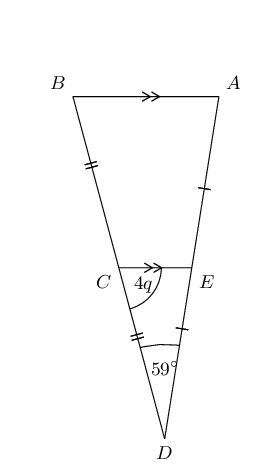Also $$\hat{A} = D\hat{E}C$$

\begin{align*} \hat{A} + 4 q + 59^{\circ} & = 180^{\circ} \qquad \text{(sum of }\angle\text{s in } \triangle \text{)} \\ \hat{A} & = 180^{\circ} - \left( 4 q + 59^{\circ} \right) \\ & = - 4 q + 121^{\circ} \end{align*}

In terms of $$q$$, the answer is: $$\hat{A} = -4q + 121^{\circ}$$.

You are now told that $$E\hat{C}D$$ has a measure of $$72^{\circ}$$. Calculate for the value of $$q$$.

\begin{align*} E\hat{C}D & = 72^{\circ} \\ 4 q & = 72^{\circ} \\ q & = 18^{\circ} \end{align*}

The figure below shows $$\triangle GHK$$ crossed by $$LJ$$. Points $$J$$ and $$L$$ bisect their respective sides of the triangle.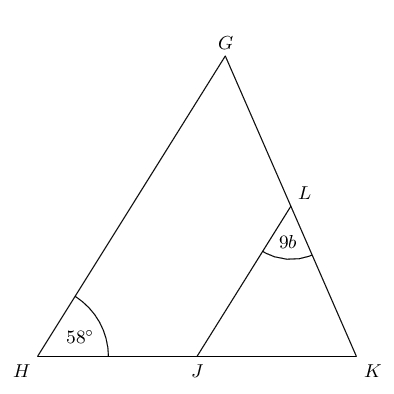Given the angles $$\hat{H} = 58^{\circ}$$ and $$K\hat{L}J = 9 b$$, determine the value of $$\hat{K}$$ in terms of $$b$$.

Using the mid-point theorem we can add the following information to the diagram: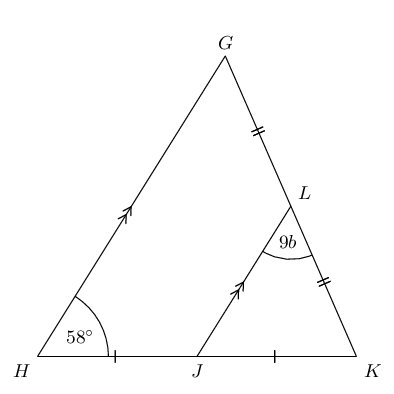Also: $$\hat{H} = K\hat{J}L = 58^{\circ}$$

\begin{align*} \hat{K} + 9 b+58^{\circ} & = 180^{\circ} \qquad \text{(sum of }\angle\text{s in } \triangle \text{)} \\ \hat{K} & = 180^{\circ} - \left( 9 b+58^{\circ} \right) \\ & = - 9 b + 122^{\circ} \end{align*}

In terms of $$b$$, the answer is: $$\hat{K} \; = - 9 b + 122^{\circ}$$.

You are now told that $$\hat{K}$$ has a measure of $$74^{\circ}$$. Solve for the value of $$b$$. Give your answer as a fraction.

\begin{align*} \hat{K} & = 74^{\circ} \\ - 9 b + 122^{\circ} & = 74^{\circ} \\ b & = \frac{16}{3} \end{align*}